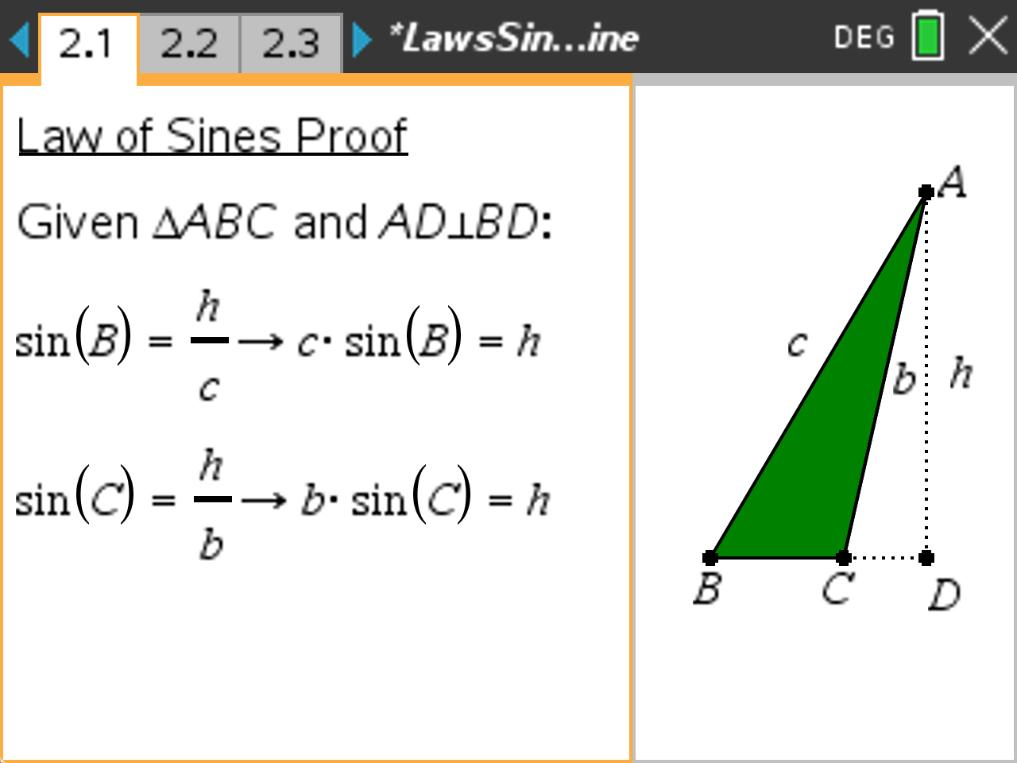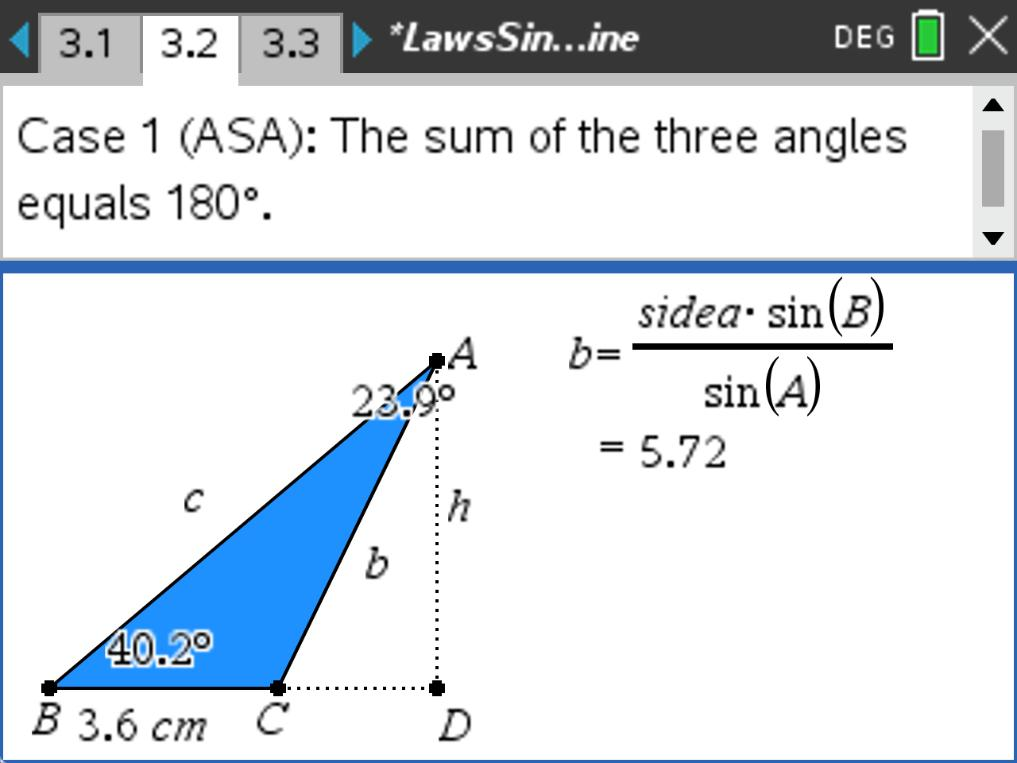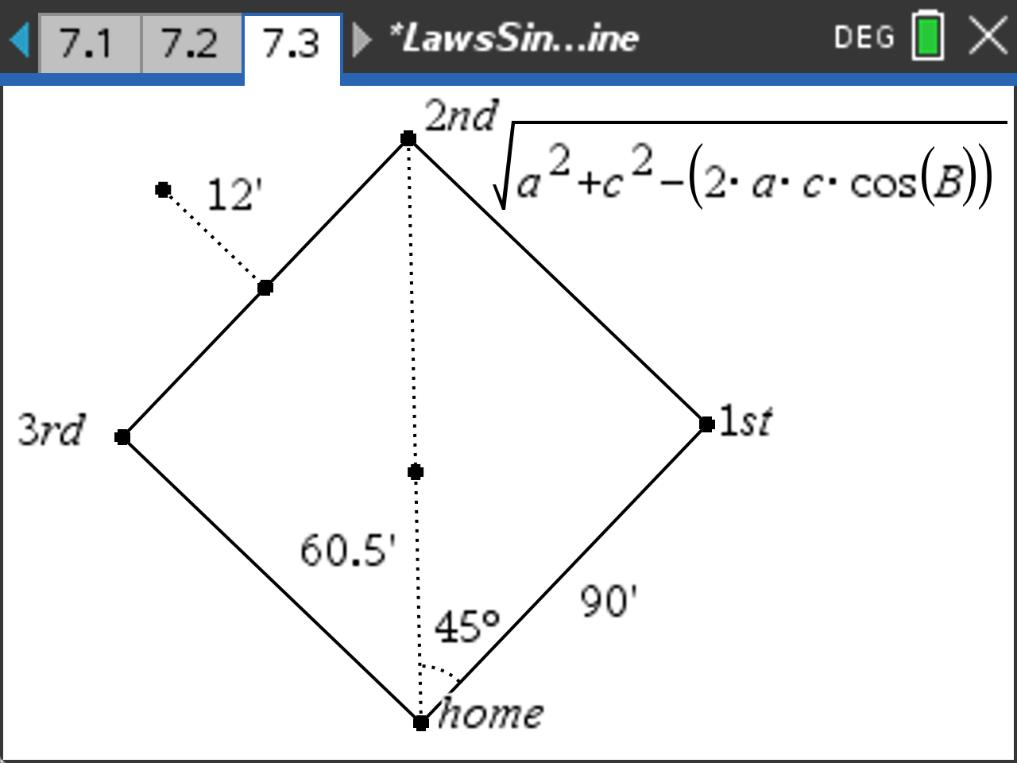### Mathematics lessons for IB® Diploma Programme

Analysis and Approaches | Geometry and Trigonometry## Law of Sines and Cosines

### Overview

Students explore the proofs of the Laws of Sine and Cosine, investigate various cases where they are utilized, and apply them to solve problems.

## Key Steps## Step 1

Problem 1 gives students the opportunity to review the Law of Sines and Cosine. They are also asked to recall from Geometry what SAS, ASA, SAA, SAS, SSS, and SSA mean and which one does not always work. In Problem 2, students prove the Law of Sine. The proof involves using right triangle trigonometry.## Step 2

Students use the Calculate tool to determine the length of a side or measurement of an angle for the various cases. In each case, students will verify the calculations using the Length or Angle tool.## Step 3

Students are given an opportunity to explore real world applications of the Law of Sine and the Law of Cosine. They can solve the problems using the Text and Calculate tools directly on the TI-Nspire document.